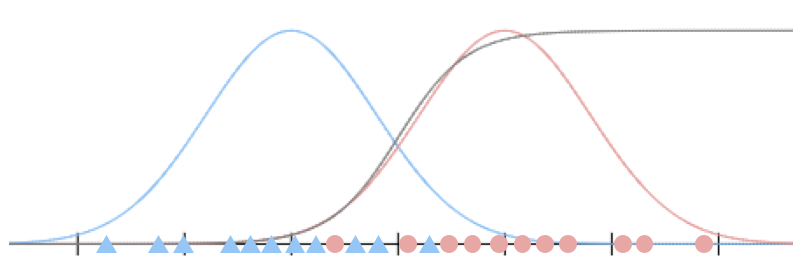## 例一：高斯判别分析和 logistic 函数

1. $p(y)$是均匀分布的，也就是$p(y=1)=p(y=0)$
2. $x$的条件概率分布（$p(x|y=0)$和$p(x|y=1)$）满足高斯分布。## 例二：垃圾邮件分类（1）

### Laplace 平滑

Laplace平滑就是来帮助解决这个问题的。

Laplace平滑中，我们会采取如下策略:

## 例三：垃圾邮件分类（2）

$x_j$表示词汇$j$在邮件中出现的次数。上述的特征向量也就是词频向量了。这种模型，我们称为多项式事件模型（Multinomial Event Model）。

$n$表示词典中词汇的数量，也就是特征向量的长度；